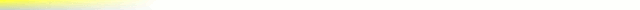# [mkgmap-dev] mkgmap r4048 seems to fix problem with hill shading

From Frank Stinner Frank.Stinner at kabelmail.de on Sat Jan 13 13:09:35 GMT 2018

```Hi Gerd,

yes, at first we have to identify the maptile. Perhaps can Minko help. Thats a "trial and error" play. Perhaps with comment out in areas.list?

You ask for the input format? It's an ordinary textfile. One text-line for one data-line. The values in a line are separated by space, comma,
semicolon or tab. If this file have q.e. 200 x 150 values, create BuildDEMFile 3 x 4 DEM-Tiles.

As next i extract with the simple perl-script extracttiles.pl a range of tiles, encode this whith

BuildDEMFile --dem="%TESTMAPDIR%\Product1\99950001\99950001.DEM" -O --data=dem.data --lastcolstd=false --left=0 --top=0.999991893768310546875
--dlon=0.0003 --dlat=0.0003

and see on the testmap 99950001.osm, what's going on. It's only "trial and error".

Frank

---
Diese E-Mail wurde von Avast Antivirus-Software auf Viren geprüft.
https://www.avast.com/antivirus
-------------- next part --------------
﻿use strict;

my \$x = 0;
my \$y = 0;
my \$x2 = 0;
my \$y2 = 0;

if (@ARGV >= 0) { \$x = shift @ARGV; }
if (@ARGV >= 0) { \$y = shift @ARGV; }
if (@ARGV >= 0) { \$x2 = shift @ARGV; }
if (@ARGV >= 0) { \$y2 = shift @ARGV; }

print STDERR "lese Daten ...\n";

my @lines = ();
while (<STDIN>) {
chomp \$_;
my \$l = \$_;
push @lines, \$l;
}

print STDERR "extrahiere Kachelbereich [\$x, \$y] ... [\$x2, \$y2]\n";

my \$xstart = \$x * 64;
my \$ystart = \$y * 64;
my \$xend = \$x2 * 64 + 64;
my \$yend = \$y2 * 64 + 64;

for (my \$j = \$ystart; \$j < \$yend && \$j < @lines; \$j++) {
my @cols = split /[\s;,]/, \$lines[\$j];
for (my \$i = \$xstart; \$i < \$xend && \$i < @cols; \$i++) {
if (\$i > \$xstart) {
print ",";
}
print \$cols[\$i];
}
print "\n";
}
-------------- next part --------------
A non-text attachment was scrubbed...
Name: 99950001.osm
Type: text/xml
Size: 1018 bytes
Desc: not available
URL: <http://www.mkgmap.org.uk/pipermail/mkgmap-dev/attachments/20180113/8e98a093/attachment-0001.xml>
```